# While loop in Python

Loops: Loops help to execute the set of code blocks repeatedly many times till the condition is met.

### Why do we need loops?

Let’s say we want to add 2 numbers which can be easily done by number1+number2

But what if there is a requirement to add 100 numbers? Well, here loops play an important role

As mentioned in the definition, the code block in the loop will run 100 times hence we’ll get the desired output, So loops are very efficient to execute the same instructions again and again.

Python has For loop, while loop

### While loop:

While loop executes a code block as far as the condition specified is true.

Note: Preferred to use while loop when the number of iterations is unknown.

Syntax: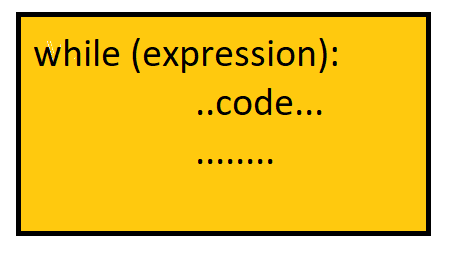### How while loop works:

1. The while loop evaluates the condition which is inside the parentheses after the “while” keyword
2. If the condition is found to be true it will execute the block of code inside the loop
3. Step 2 will occur again and again till the condition is false
4. If the condition is false control will come out of the loop.

Note: Indentation for every line of code in the while loop should be equal and it’s mandatory to give proper indentation.

Flow chart: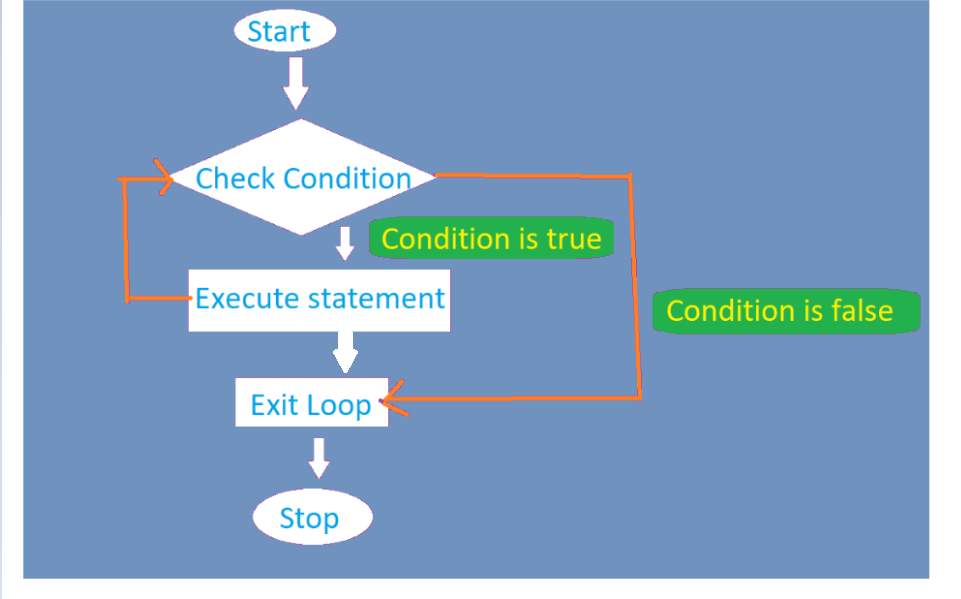Let’s see an example of how the while loop works internally:

Printing sum of first n numbers: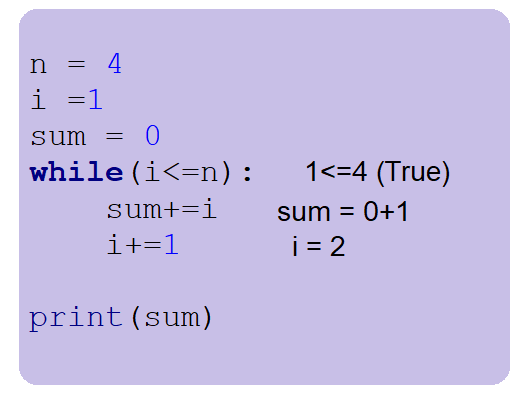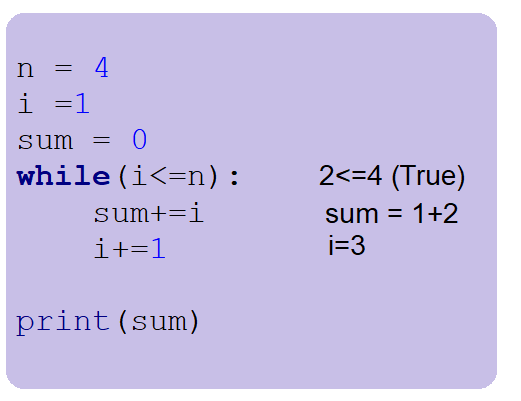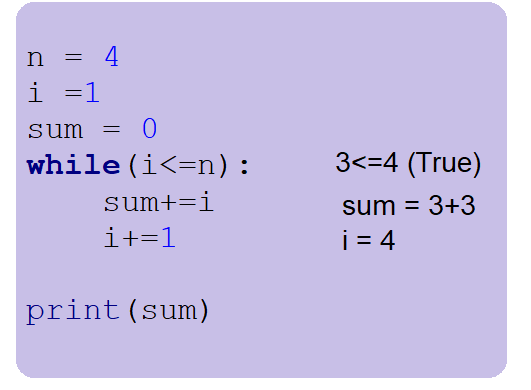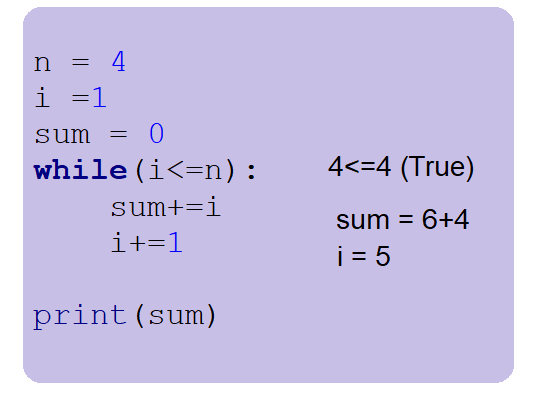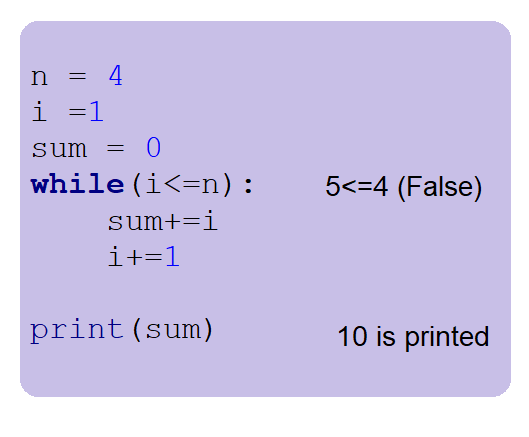Code:

## Python Code

``````n = 10
i =1
sum = 0
while(i<=n):
sum+=i
i+=1

print("Sum of first",n,"numbers is", sum)``````

Output: Sum of first 10 numbers is 55

While loop with else:

While loop can have an else statement, this else statement will be executed when the condition is false in the while loop, and else will not be executed when a loop is terminated with a break

Program to demonstrate while loop with else ( without break)

Code:

## Python Code

``````i =1

while(i<=4):
print(i)
i+=1
else:
print("Else block executed")
``````

Output:

1
2
3
4
Else block executed

Explanation:

The loop is not terminated by “break”, and it is terminated because the condition met false, So the else part is also executed.

Program to demonstrate while loop with else ( with break)

Code:

## Python Code

``````i=1
while(i<=4):
print(i)
if i == 3:
break
i+=1
else:
print("Else block executed")
``````

Output:

1
2
3

Explanation: Since the loop is terminated by “break”, so the else part will not be executed.

Exercise for you:

1. Print numbers from n to 1 using the while loop
2. Find the multiplication of the first 10 numbers using the while loop